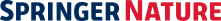# A nonparametric multiple imputation approach for missing categorical data

Posted on 2017-06-06 - 05:00
Abstract Background Incomplete categorical variables with more than two categories are common in public health data. However, most of the existing missing-data methods do not use the information from nonresponse (missingness) probabilities. Methods We propose a nearest-neighbour multiple imputation approach to impute a missing at random categorical outcome and to estimate the proportion of each category. The donor set for imputation is formed by measuring distances between each missing value with other non-missing values. The distance function is calculated based on a predictive score, which is derived from two working models: one fits a multinomial logistic regression for predicting the missing categorical outcome (the outcome model) and the other fits a logistic regression for predicting missingness probabilities (the missingness model). A weighting scheme is used to accommodate contributions from two working models when generating the predictive score. A missing value is imputed by randomly selecting one of the non-missing values with the smallest distances. We conduct a simulation to evaluate the performance of the proposed method and compare it with several alternative methods. A real-data application is also presented. Results The simulation study suggests that the proposed method performs well when missingness probabilities are not extreme under some misspecifications of the working models. However, the calibration estimator, which is also based on two working models, can be highly unstable when missingness probabilities for some observations are extremely high. In this scenario, the proposed method produces more stable and better estimates. In addition, proper weights need to be chosen to balance the contributions from the two working models and achieve optimal results for the proposed method. Conclusions We conclude that the proposed multiple imputation method is a reasonable approach to dealing with missing categorical outcome data with more than two levels for assessing the distribution of the outcome. In terms of the choices for the working models, we suggest a multinomial logistic regression for predicting the missing outcome and a binary logistic regression for predicting the missingness probability.

## CITE THIS COLLECTION

or
Select your citation style and then place your mouse over the citation text to select it.

## Usage metrics## AUTHORS (4)Muhan ZhouYulei HeMandi YuChiu-Hsieh Hsu